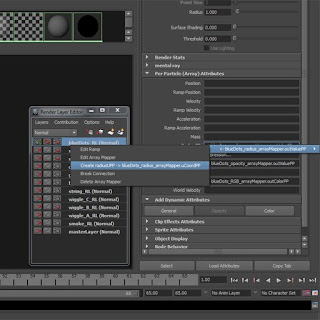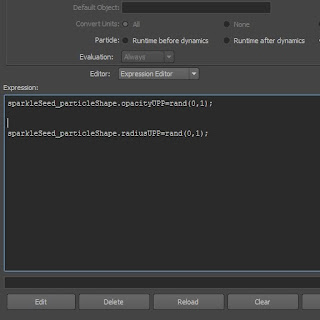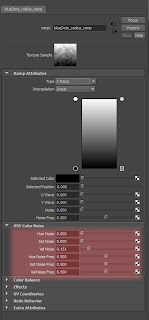## Thursday, 28 April 2011

### Using multiple emFly compounds

When using Eric Mootz's excellent emFly system, it is possible to use multiple emFly compounds.

Let's say you have a complex flight plan and you want two different landing behavours, you will need to use a unique emFly compound for each landing.

Using ICE States, you can plug in a new emFly compound into each State's 'execute once enter' and 'execute every frame'.I haven't seen this documented anywhere, but it worked for me in Softimage 2011.

Hey, my first Softimage ICE post !

## Tuesday, 19 April 2011

### nParticle cache creation - use forward slash

When you want to create a nParticle cache in a custom folder, say c:\particles,
enter that folder in the options box, but use the forward slash instead.I'm not sure if this is a bug, but I found that using a backslash will not work.

## Friday, 15 April 2011

### Adding variation to per particle attributes that use ramps

Let's say you have a classic particle (not an nParticle) which will use a ramp to assign it's radiusPP.
You want to add some variation to the ramp but have each particle read a unique value and then travel up the ramp over it's lifespan as usual.

Here are the steps:

2. Create the ramp by right-clicking in the RadiusPP box4. Once you have the RadiusUPP attribute, give it a random value betweek 0 and 1 via the expression editor5. To link the UPP value to the ramp, we need to re-make the array mapper. Right-click on the RadiusPP box and delete the arrap mapper (you will want to rename or check the name of the ramp before you do this)

6. Right-click in the RadiusPP box again and click on the Create Ramp options box. Choose RadiusUPP for the input U, leave input V as Particle's Age and then choose the ramp in the Map To list.Now each particle is going to read a value at a random point across the bottom of the ramp and then move up the ramp over it's lifetime. Now we need to add some variation to the ramp. A quick way to do this is to use the ramp's built in noise functions## Saturday, 9 April 2011

### Approximating ConservePP

Wouldn't it be nice sometimes if we could have a per-particle Conserve attribute? Well, a reasonable approximation can be to use the mass attribute instead.

The conserve attribute essentially is a multiplier on the particle's velocity. For example, if a particle has velocity 100 at frame 1 and a conserve of 0.5, then a frame 2 the velocity would be 50, at frame 3 it would be 25, and so on.

So, the conserve attribute acts as a brake on the velocity.

In the physical world, mass (specifically Inertial mass) can be defined as 'resistance to acceleration'. When simulating particle dynamics we can use mass as a dampening effect on the motion of a particle, in a similar way that conserve does. The main difference is that we can define the mass on a per-particle basis: the higher the mass the higher the resistance to motion and the lower the 'conservePP' value we are creating.

## Friday, 8 April 2011

### Gravity versus Uniform field

In nature, a body is affected by gravity in relation to its mass. Newton's equation of gravity show this:

F= G (M * m) / r^2

Where M and m are the masses of the bodies affected.

Also, Newton's laws of motion (he was a clever guy) show that force is also proportional to mass:

F = m a

If we equate the two forces we see that both sides have the mass, m, which cancel each other out.

So, the acceleration that the body experiences is not proportional to its mass.

This is not true for a Universal force. The strength of a universal force is not linked to the mass of the body and so when we equate it with the law of motion we get something like this:

m a = U

Where U is the arbitrary universal force.

So the acceleration experienced by the body is now (inversely) proportional to its mass.

Maya copies nature in this regard, so if you want to use variable masses to drive your simulations, be aware that the Gravity force will affect all the particles uniformly but a Uniform field will affect them in (inverse) proportion to their mass.